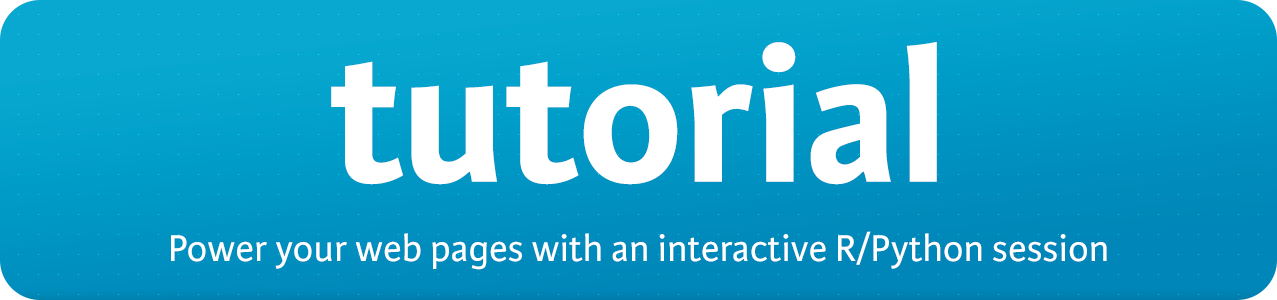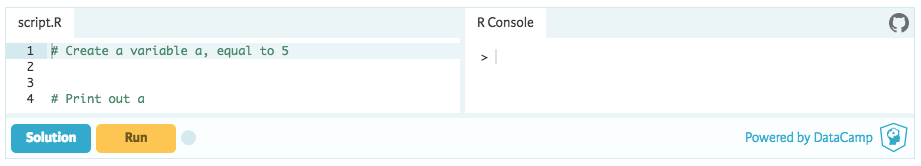# README.md In tutorial: Convert R Markdown Files to DataCamp Light HTML Files`knitr` utility to convert your static code chunks into an R editor where people can experiment. Powered by DataCamp Light, a lightweight version of DataCamp's learning interface.

## Installing the package

Latest released version from CRAN

``````install.packages("tutorial")
``````

Latest development version from GitHub:

``````if(!require(devtools)) {
install.packages("devtools")
}
devtools::install_github("datacamp/tutorial")
``````

## Getting started

Add the following chunk at the top of your R Markdown document

`````````{r, include=FALSE}
tutorial::go_interactive()
```
``````

Knit your document: in RStudio, simply hit "Knit HTML", or use

``````rmarkdown::render("path_to_my_file", output_format = "html_document")
``````

## How it works

R vignettes, blog posts and teaching material are typically standard web pages generated with R markdown. DataCamp has developed a framework to make this static content interactive: R code chunks are converted into an R-session backed editor so readers can experiment.

### Fiddles

If you render an R Markdown document like this:

``````---
title: "Example Document"
output:
html_document:
self_contained: false
---

```{r, include=FALSE}
tutorial::go_interactive()
```

By default, `tutorial` will convert all R chunks.

```{r}
a <- 2
b <- 3

a + b
```
``````

An HTML file results that features an in-browser R editor with a session attached to it, where you can experiment.Notice that the option `self_contained: false` is used. That way, always the latest version of the DataCamp Light JS library is fetched when the document is opened. If `self_contained: true`, the version of the JS library at 'knit time' is baked into the HTML document. This can cause backwards compatibility issues.

### Coding challenges

You can also embed coding challenges into your webpages. This group of code chunks:

`````````{r ex="create_a", type="pre-exercise-code"}
b <- 5
```

```{r ex="create_a", type="sample-code"}
# Create a variable a, equal to 5

# Print out a

```

```{r ex="create_a", type="solution"}
# Create a variable a, equal to 5
a <- 5

# Print out a
a
```

```{r ex="create_a", type="sct"}
test_object("a")
test_output_contains("a", incorrect_msg = "Make sure to print `a`.")
success_msg("Great!")
```
``````

Converts to the following DataCamp Light exercise:### Vignettes

You can power your package's vignettes with DataCamp Light by adding the same line to the top of your vignette:

``````---
title: "Tutorial Basics"
author: "Filip Schouwenaars"
date: "`r Sys.Date()`"
output: rmarkdown::html_vignette
vignette: >
%\VignetteIndexEntry{Tutorial Basics}
%\VignetteEngine{knitr::rmarkdown}
%\VignetteEncoding{UTF-8}
---

```{r, include=FALSE}
tutorial::go_interactive()
```

_remainder of vignette omitted_
``````

## Python

You can also code up Python exercises with `tutorial`:

``````---
title: "Example Document"
output:
html_document:
self_contained: false
---

```{r, include=FALSE}
tutorial::go_interactive()
```

Here's an example of a Python fiddle/

```{python}
a = 2
b = 3

print(a + b)
```
``````

## Other Documentation

• Tutorial Basics Vignette: explanation on how to convert your static R code chunks into interactive fiddles or exercises, where you can also experiment with DataCamp Light itself.
• R Markdown and knitr for dynamic documents with R.
• DataCamp Light JS library
• Course creation for DataCamp. The documentation includes information on how to get started with course creation, what the different components of an exercise are, how you can write Submission Correctness Tests (SCTs) etc.

For more details, questions and suggestions, you can contact content-engineering@datacamp.com.

## Try the tutorial package in your browser

Any scripts or data that you put into this service are public.

tutorial documentation built on May 2, 2019, 9:34 a.m.